# LORE #4: Complete Time-Series Project for Stock Price Forecast on RStudioSometimes simple questions need complex insights. This project brings one of the most popular tools in statistics into a financial context, while providing insight towards the potential of more in-depth analysis.

# Personal Reflection: A Real Scenario, An Imaginary Client

Cue the intro.

As of early February 2019, I am currently sitting inside a research centre in the University of British Columbia Sauder School of Business independently conceiving ways to convert my combined scientific statistics degree into a role as a financial analyst.

In college, the hardest of science courses always started at general basics before narrowing down into complex concepts. Upon completing my degree, I gained the basics from online courses and CFA lectures, but going deeper into applying knowledge and seeing complex concepts was difficult without a syllabus. Reading financial news was not enough.

After camping out at the research centre for days on end thinking how complicated the data from a Bloomberg Terminal was, an idea sparked. Learning financial models is like learning statistical models.

Scientific labs have tools like transmitted electron microscopy to view the nuclei of neurons, and financial research centers have tools like Bloomberg Terminals and Capital IQ monitors to view financial data. The parallel is that financial and scientific research alike uses a combination of contextual interpretation and quantitative models to reach answers that would be of value to specific audiences. Learn the models, learn the context, and then link them.

This re-framed my way of thinking by creating a vision that I could work towards. With my limited knowledge today, I at least have enough imagination to reword the intro into something worth more.

As of early February 2020, I am currently sitting at my office desk at a data analytics firm. The analytics team was approached by a risk-averse client seeking insight towards the stability of tech stocks. Before developing strategies for portfolio management, the team wants to do a quick analysis on stock values for the major players in the industry. I am tasked with forecasting the value for Amazon.

Within two weeks, I was able to build upon my coursework knowledge of regression analysis into a financial context that could be of use to a quantitative research team.

# Background Research and Model Choice

Amazon is a multinational technology company focusing in e-commerce, cloud computing, and artificial intelligence in Seattle, Washington. It is one of the Big Four or “Four Horsemen” of technology along with Google, Apple and Facebook due to its market capitalization, disruptive innovation, brand equity and hyper-competitive application process. News for Amazon, and its CEO Jeff Bezos, is reported almost every hour as the company continues to impact multiple industries and expand its services.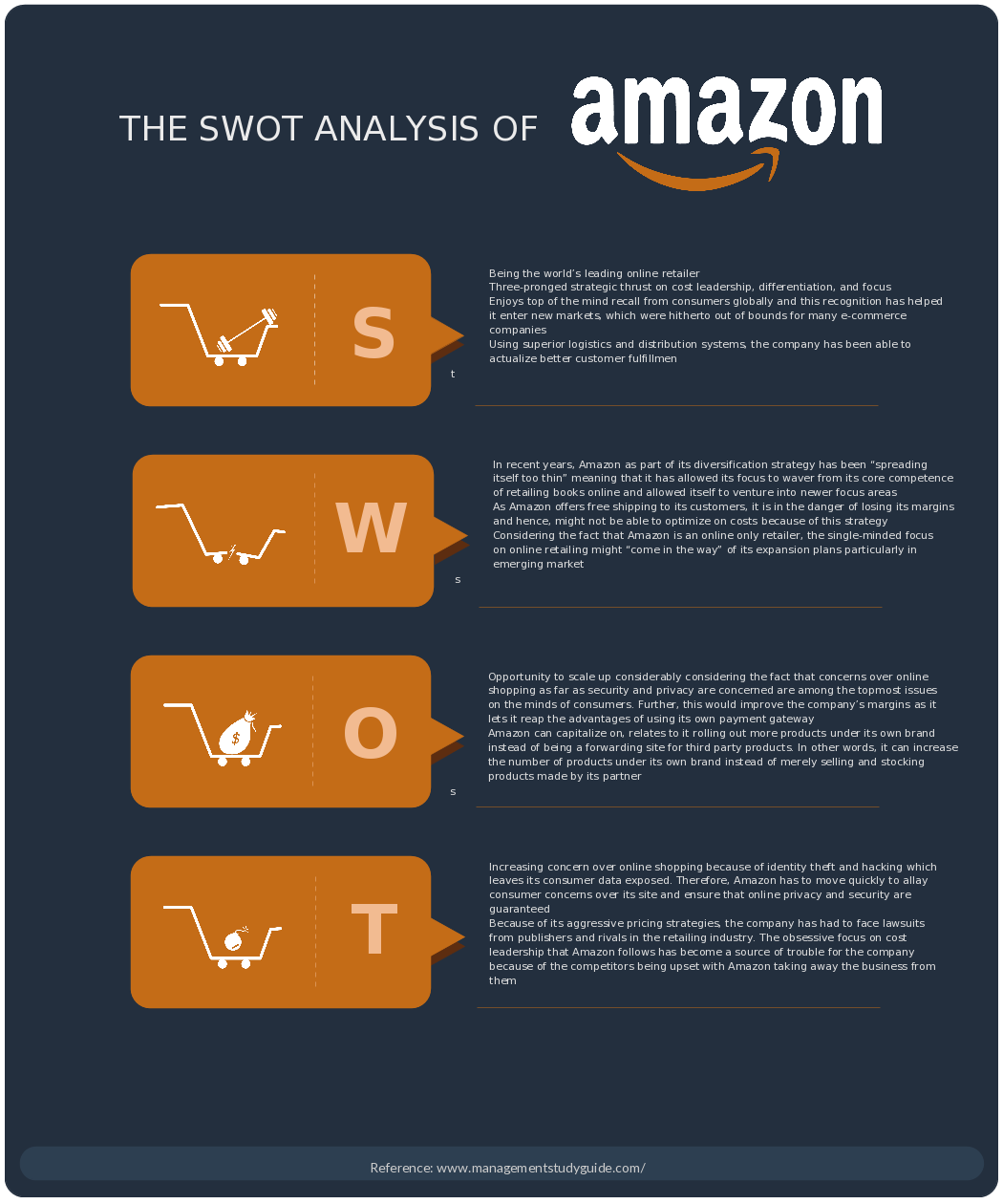It is clear that the “Amazon Effect” has shown the company stands tall over the retail industry, online or not. Read more: https://www.entrepreneur.com/article/325556

Using the stock values for Amazon, the team wants to know if the stock value would retain its growth based on its previous values. As stock values change with time and are affected by other indicators that may or may not substantiate its real value, we can use a time-series model that examines differences between values in the series instead of actual values. This scenario falls perfectly in line with regression analysis, or more specifically, an ARIMA model.

# What is the ARIMA model?

ARIMA stands for Auto Regressive Integrated Moving Average. As explained in the paragraph above, ARIMA forecasts short-term future values based on the increasing or decreasing change in historical values, or inertia.

Also known as the Box-Jenkins model (after the original authors), ARIMA requires two things: at least 40 historical data points (or stock values in this case) and a stable or consistent pattern over time with few outliers.

Formally, ARIMA combines three statistical components to form a (p,d,q) notation.

• AutoRegression (p) — Using a weighted sum of past values, a regression equation is created to describe data points of the time series data regressed on their own lagged values. Unlike multiple regression which forecasts a variable based on a linear combination of predictors, autoregression uses a combination of past values of a single predictor.
• Integration via Differences (d) — Since ARIMA requires a stable or consistent pattern, the time series should be transformed from non-stationary into stationary, or differenced, in order to eliminate trends. Differencing subtracts raw data observations in the current period from the previous ones until the data does not grow at an increasing rate. This component also removes seasonal trends.
• Moving Average (q) — From the differenced data, a trend-following, or lagging, indicator is created that helps determine the odds of upward or downward trends. The longer the time period for the moving average, the greater the lag, the more likely the change. Moving averages are represented by the number of error terms included in the regression equation.

So the basic goal in mind is to grab data from a proper source, find the ARIMA notation, and fit a model based on the ARIMA notation for a specific purpose, and then validate the model.

# Summary of Procedure

This end-to-end project seeks to carry out the full scope of ARIMA forecasting. We perform multiple statistical checks on different forms of stock returns and program a flexible ARIMA model that can be easily used for any stock.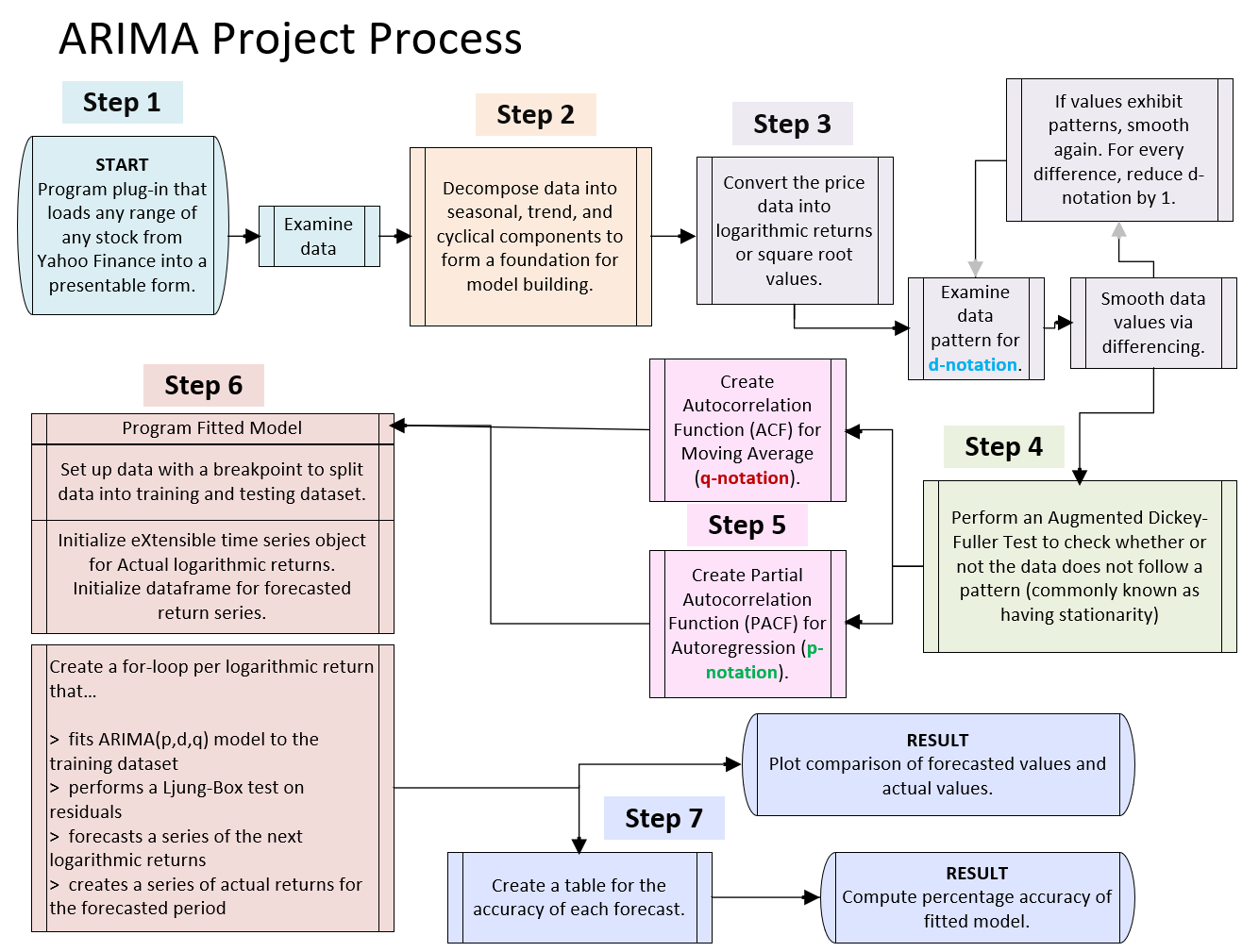The following flowchart, created by Microsoft Visio, shows the process taken to create the ARIMA model application used for this project. This blogpost sections the steps in order to reach the results of the model.

Code for all steps in this article is presented as images, which means the lines cannot be directly copied and pasted.

For R source code of the entire project in a single file, along with comment descriptions, feel free to email me at aaronjyen@gmail.com.

# Step 1: Loading and Graphing Datasets

The following code pulls any stock and any range from Yahoo Finance, one of the most popular public resources for stock data. The 5 R packages loaded are able to directly use information from the online database, so long as wifi is available. Without changing the variable names of specific parts of the code, the strings in lines 9–11 can be changed for flexible use.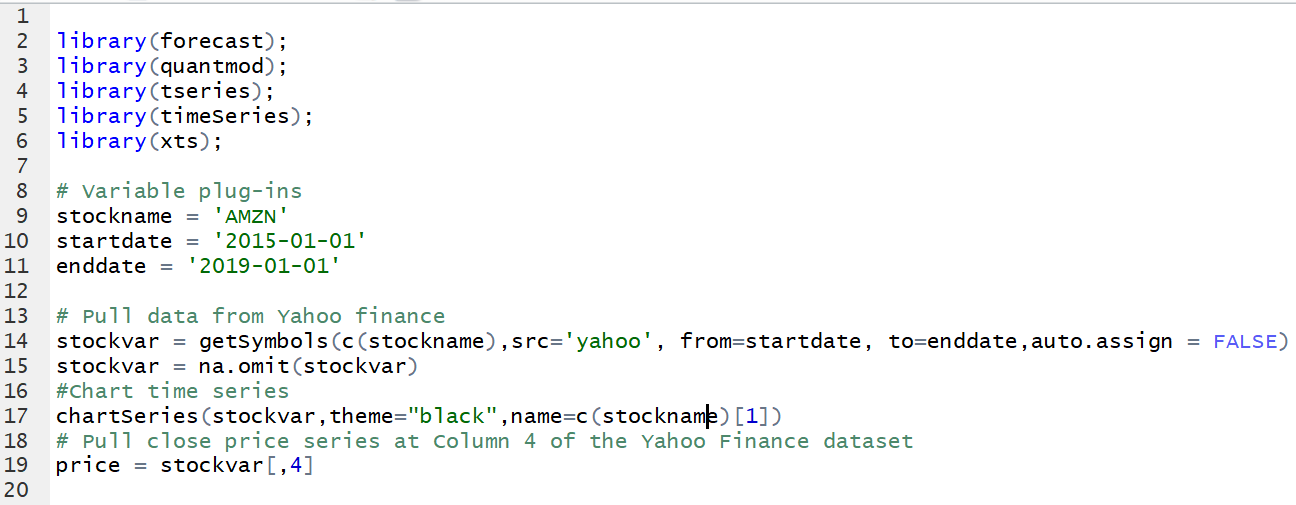When installing and running packages, make sure that the packages include dependencies.

Since we are pulling data from Yahoo Finance directly and considering a stock that is updated regularly, we can eliminate a couple of steps for data preprocessing or cleaning, converting Excel file data into indexes, or accounting for empty values (aside from Line 15).

Line 17 graphically represents the high and low dollar-value fluctuations in weekly close prices of the AMZN stock, along with the volume of shares traded during each period of time.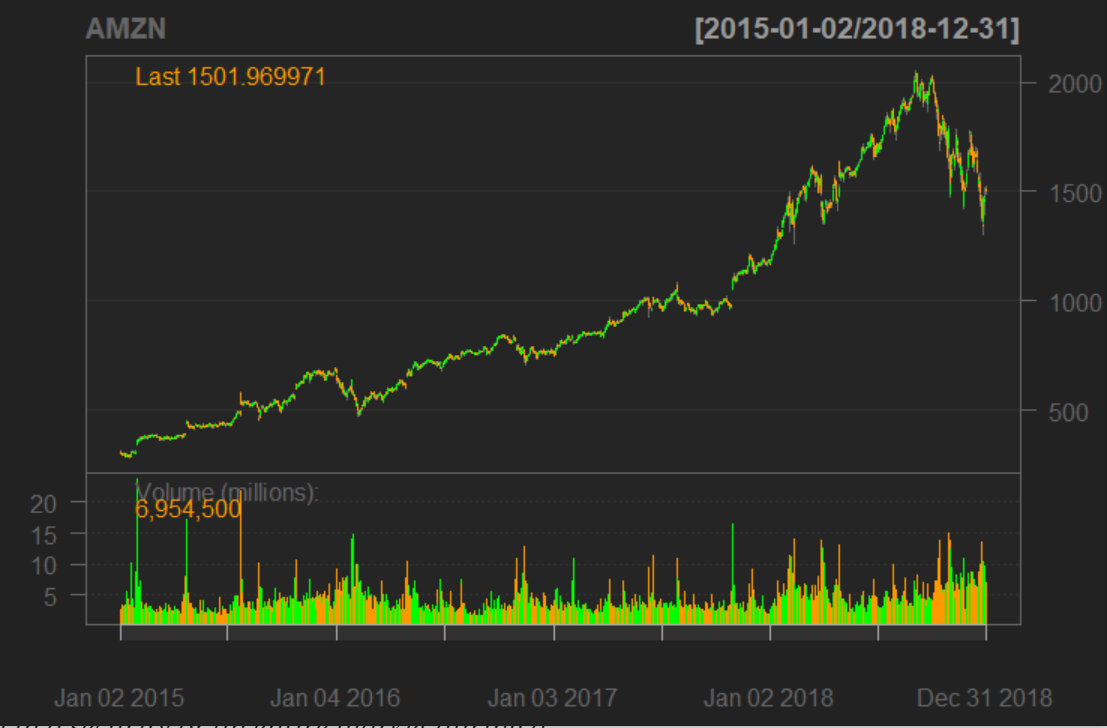This is the black theme of the chartSeries() command.

For this analysis, we are isolate weekly close prices as the benchmark for future predictions, as those values are assumed to best reflect the changes in real values of the stock during the time frame. When looking at the historical data values for AMZN, the weekly close prices are in the 4th column, which leads to Line 19.

## Observations From Examining The Graph

Upon briefly looking at the data, we can see that the stock value increased over time since 2015, but spiked during 2018. There is no apparent pattern that can be used to scale the value of the stock price because the trends are not linear, and there is no mathematical formula to describe the change in curve or the fluctuations between increasing or decreasing prices.

As the stock price spikes in 2018, the variance between datapoints also appears to increase, with stock prices being especially volatile at the most recent dates.

# Step 2: Decomposing The Data Into Components

To further analyze trends in the data, we can decompose the data into seasonal, and trend components. Decomposition is the foundation for building the ARIMA model by providing insight towards the changes in stock fluctuations.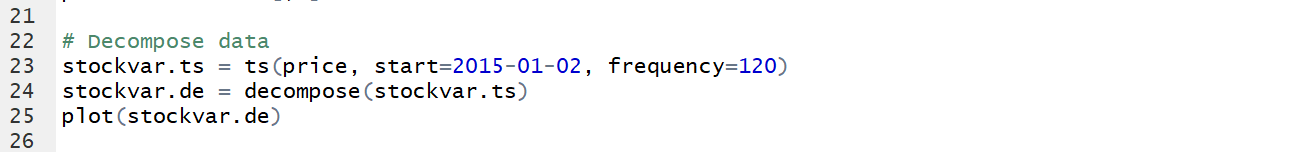The decompose() command in R conveniently compiles all of the trends together for visual representation. Mathematical representations would require coding for individual components.
• The observed data section is a reproduction of the data from Step 1 for comparison. The observations are explained above.
• The trend component describes the overall pattern of the series over the entire range of time, taking into account increases and decreases in prices together. From the plot above, the trend is overall increasing.
• The seasonal component describes the fluctuations in stock price based on the calendar or fiscal year. From the plot above, the peak in stock price occurs every year at Q4 (July, August, and September) and the trough in stock price occurs every year at Q2 (January, February, and March), with clear oscillating fluctuations in between.
• The random (residual) error, or noise section describes the trends that cannot be explained by trend or seasonal components. Statistically, these errors are the difference between the observed price and the estimated price. Random error is particularly important for this project because a statistical model can only be fit if the residuals are independent and independently distributed (more will be explained in Step 6). One notable observation is that from mid-2018 to 2020, the plot shows more fluctuation, meaning there is greater variance and greater statistical error. This means more recent and future points become more unpredictable, as shown in the spike in stock price from Step 1’s plots.

From Step 2, we gather that there is a clear trend that we can observe from each of the components of the data. Based on the definition of an ARIMA model, this data indeed has a stable or consistent pattern over time with few outliers. However, since the random (residual) error is greater with more recent datapoints, it would be a good idea to reconfigure the data to fit a regression that accounts for the residuals.

# Step 3: Smoothing The Data

To stabilize variance, we extract the logarithmic returns and the square root values from the prices.

There are many reasons to use logarithmic returns, but in short, the fluctuations in prices transformed into returns can be better compared over time and used to describe trends. The result is a smoothed curve with reduced variation in the time series, so a forecasting model can fit more accurately.

Square root values instead of raw prices are used to scale the volatility between points to manage the time horizon of the stock. This is especially important because the longer a position is held, the greater a potential loss can be found.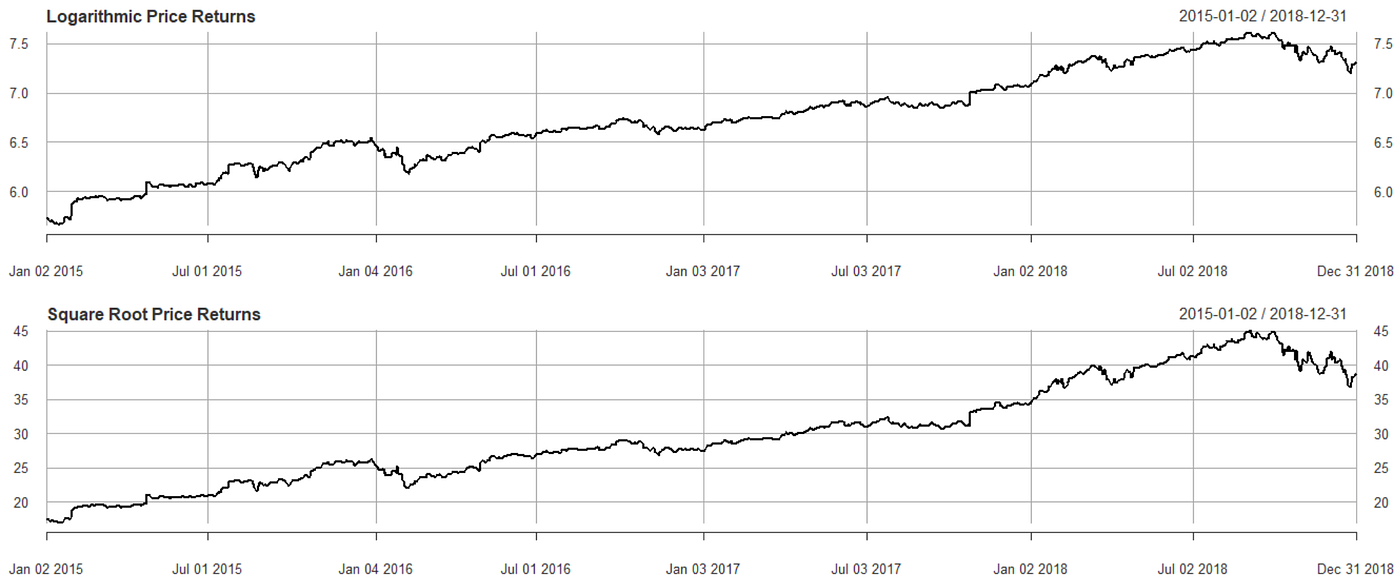The time range (x-axis) of the datapoints are adjusted to weekly values across a 3-year period, which is of particular importance, since the residual error is observed to be greatest in this range.

When viewing the graphs, the range of values of the y-axis are much smaller than the range of values in the observed data. In comparison to the fluctuations seen in the raw data in Steps 1 and 2, the transformed data plots are more linear.

To smooth the data even more, we want to remove the fluctuations in data altogether. As a requirement of the Integration part of the ARIMA model, we difference the logarithmic returns and the square root values to turn the data into its stationary form.

Stationarity in data is useful for forecasting models because an analyst can simply conclude from prediction that a plot’s trends will be the same in the future as they have been in the past.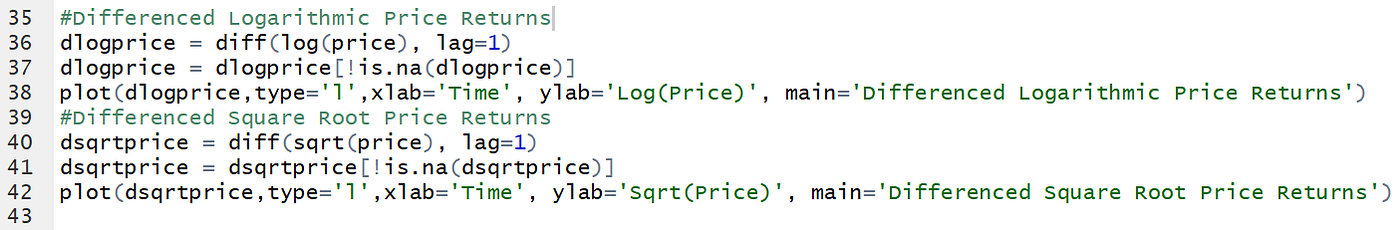Line 37 and 41 account for null values that could occur from the dataset.

In this project, we only difference once. Differencing to the first order does the following:

• Statistical properties, such as mean, variance and autocorrelation, are constant over time.
• Linear properties, such as y-intercept and slope, are constant over time.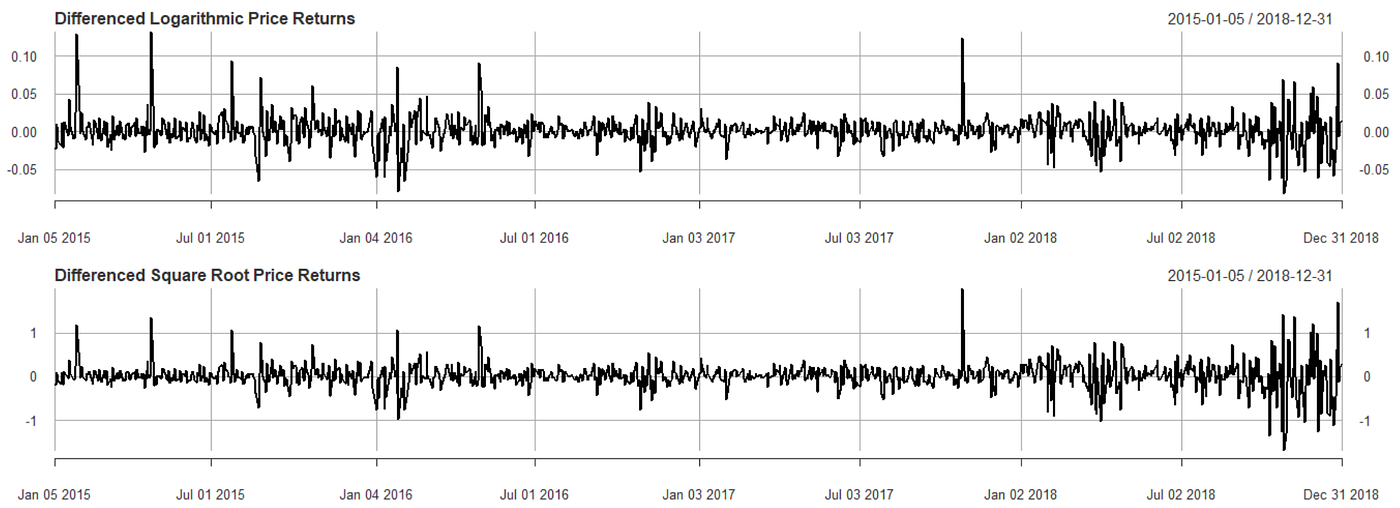The d-notation for the ARIMA model is the number of non-seasonal differences needed for stationarity. Since logarithmic returns and square root values, though scaled, may still contain seasonal trends, we do not count the differences done here as part of the notation. Therefore, the d-notation is 0 for both datasets.

Again, viewing the y-axis of the plots, the transformed data is now oscillating around 0. The deviations from 0 are much smaller than in the previous plots, meaning the data has been smoothed more. The fluctuations from these plots should be best for describing trends in the value of the stock. Confirming the observations in Step 2, most major fluctuations are found in 2018, which was when the stock returns (and price) changed the most.

If there were still fluctuations in the data, differencing a second time, or to the second order, would smooth the data further by accounting for the quadratic (curve) differences between datapoints.

# Step 4: Performing The ADF Test

We now have the graphical evidence to use the data, but before we fit an ARIMA model, we need to confirm that the data is actually usable.

The Augmented Dickey Fuller Test determines whether a unit root, a feature that can cause issues in statistical inference, is present in a time-series sample. With ARIMA model, the hypotheses in this context are to confirm stationarity, as we saw before.

For clarity…

• Ho: The time-series data includes a unit root, and is non-stationary. The mean of the data will change over time.
• Ha: The time-series data does not include a unit root, and is stationary. The mean of the data will not change over time.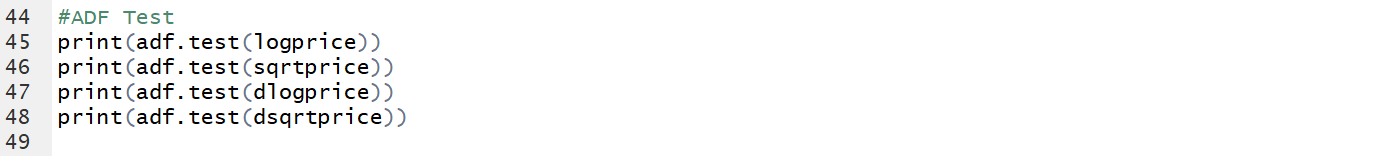Conveniently, the tseries package in R allows for adf.test() to spit out results within a single line.

The results here show what types of data would be appropriate for an ARIMA model. The Dickey-Fuller statistic shows that the more negative the number, the stronger the null hypothesis rejection, meaning there is no unit root. Similarly with any hypothesis test, the small p-value means there is strong evidence against the null hypothesis, meaning there is no unit root.

As such, to properly use an ARIMA model, we should use the differenced logarithmic returns and square root values only.

# Step 5: Creating Correlograms

Now we can move into creating the ARIMA model with the proper data prepared. In other words, we want to find the ARIMA(p,d,q) notation.

Correlograms, or autocorrelation plots, indicate how the data is related to itself over time based on the number of periods apart, or lags.

ARIMA models integrate two types of correlograms, each allowing us to determine parts of the ARIMA notation:

• the AutoCorrelation Function (ACF) displays the correlation between series and lags for the Moving Average (q) of the ARIMA model
• the Partial AutoCorrelation Function (PACF) displays the correlation between returns and lags for the Auto-Regression (p) of the ARIMA model

Most values on correlation plots range from -1 to 1. A value close to -1 implies a strong negative correlation, or an opposite effect. A value close to 1 implies a strong positive correlation, or a tandem effect. A value close to 0 implies no correlation.

As for autocorrelation plots, the blue-dotted line determines the strength of correlations. The values that cross the blue-dotted lines determine the notation for the ARIMA model for each dataset.

Upon viewing the ACF plot for the logarithmic returns, the cutoff for strong correlations (where values no longer cross the blue dotted line) is at Lag 2, which means the p-notation is 2.

Upon viewing the PACF plot for the logarithmic returns, the cutoff for strong correlations (where values no longer cross the blue dotted line) is at Lag 2, which means the p-notation is 2.

Alongside the results of Step 3, where the d-notation is 0, the model to fit onto the logarithmic price returns is ARIMA(2,0,2).

Upon viewing the ACF plot for the logarithmic returns, there is no cutoff for strong correlations (where values no longer cross the blue dotted line) since Lag 1 does not have a strong correlation, which means the p-notation is 0.

Upon viewing the PACF plot for the logarithmic returns, the cutoff for strong correlations (where values no longer cross the blue dotted line) since Lag 1 does not have a strong correlation, which means the p-notation is 0.

Alongside the results of Step 3, where the d-notation is 0, the model to fit onto the logarithmic price returns is ARIMA(0,0,0). This particular ARIMA model represents white noise, which means no model will be able to fit the square root values for this stock.

# Step 6: Programming A Fitted Forecast

Now that we are down to fitting an ARIMA(2,0,2) model for the differenced logarithmic price returns, we can build a forecast.

To set up the model, we format the data to compare real returns with forecast returns. The real returns are formatted as an eXtensible Time Series, or xts, which lets us manipulate the data as a function of time. The forecast returns are formatted as a dataframe, which lets us slot in the results from the forecast model.

The for-loop below is the working model that forecasts returns for each datapoint, and returns a time series for both real and forecast values. A brief description of each part is below.

Line 65 splits the dataset into a training and testing set by introducing a breakpoint. The training set is used to fit the parameters set by the ARIMA(2,0,2) model for estimates. The testing set is used to assess the performance of the model by providing an unbiased evaluation of its fit.

In other words, the training set is the basis for our results, and the testing set is where we determine forecasts.

Line 68 and 69 segments the training and testing sets. Each of the testing datapoints are ahead of the training datapoints.

Line 71 and 72 form the basic fitting process for the ARIMA model. The ARIMA(2,0,2) notation is entered into the arima() command for each datapoint in the training set.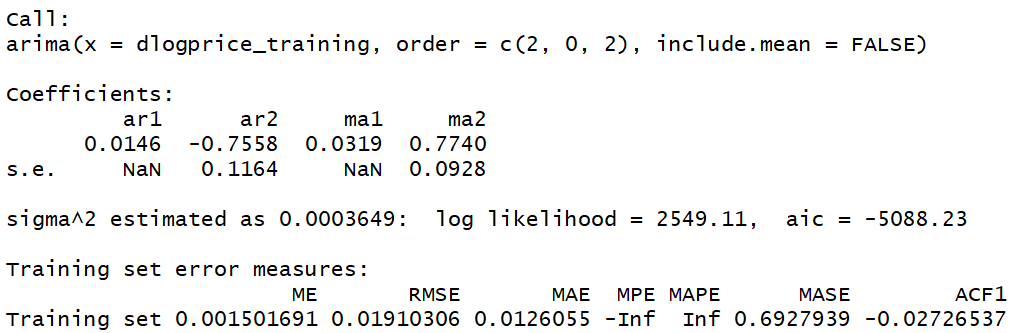This is the result of running Line 72 for the stock. This is the ARIMA model for the training set, which confirms that a fit does exist. The Akaike Information Criterion, or AIC, which measures relative amount of information lost by a given model, is very negative, which is also a good sign.

From the coefficients, we have an ARIMA(2,0,2) equation for the in-sample forecast of the data, which describes each datapoint in the sample, fitted one step ahead without residuals.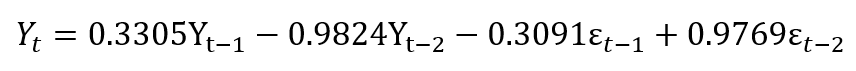The first two terms represent the AR part of the equation, which is the linear combination of observable values. The last two terms represent the MA part of the equation, which is the linear combination of the unobservable white noise disturbance terms.

Line 74 takes a one-day-ahead forecast of each datapoint of the fitted ARIMA model for the differenced logarithmic returns of the next day.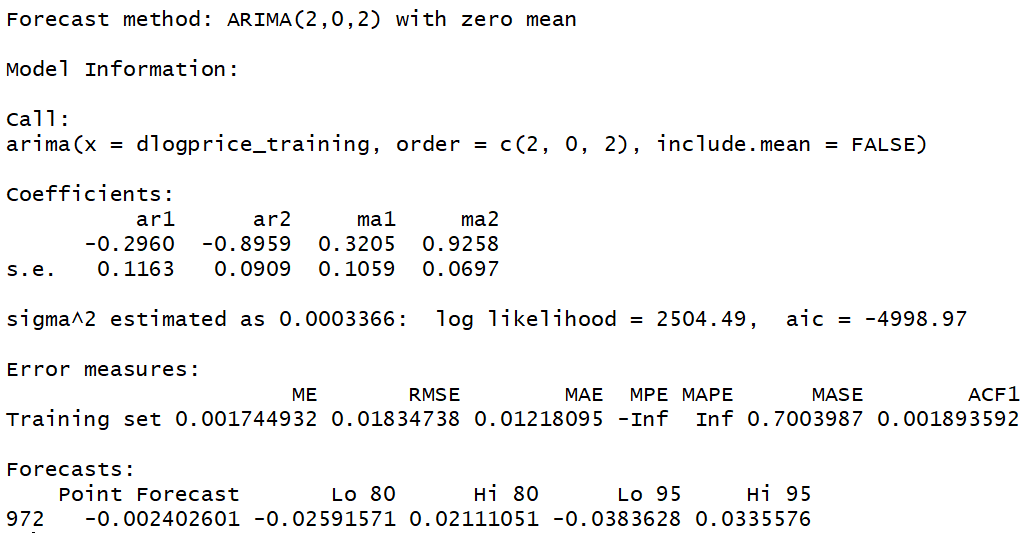Similarly, we have coefficients that form an ARIMA equation. Additionally, we have point forecast information based on 80% and 95% confidence intervals.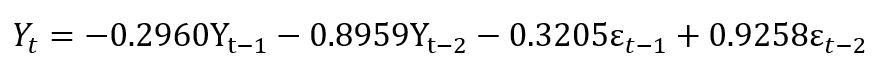This is the important equation for our forecast!

Line 77 is a Box-Ljung Test, which tests the overall randomness, or lack of fit, of each residual on a group of lags in the data.

This test can work within the for-loop because it is a portmanteau test, where the null hypothesis is specified but the alternative hypothesis is loosely defined.

For clarity…

• Ho: The data are independently distributed, relatively random, and should fit properly into the model.
• Ha: The data are not independently distributed, serially correlate, and should not fit properly into the model.

For every datapoint that does not pass the Box-Ljung Test, the loop issues a warning. The number of warnings helps strengthen our interpretation of the model and confirm validity that the trends are meaningful.

Lines 79 to 83 format the data for comparison to match the definitions set at the beginning of Step 6 by appending each return into their respective real and forecast series.

We now have all the information we need in the proper formats for comparison.

# Step 7: Visualizing And Validating The Model Results

What’s left in this project now is to present the findings for interpretation and to determine how powerful the model is at creating results.

The following code extracts the real and forecast series for the testing sets where we find our main results and plots both of them for a visual comparison.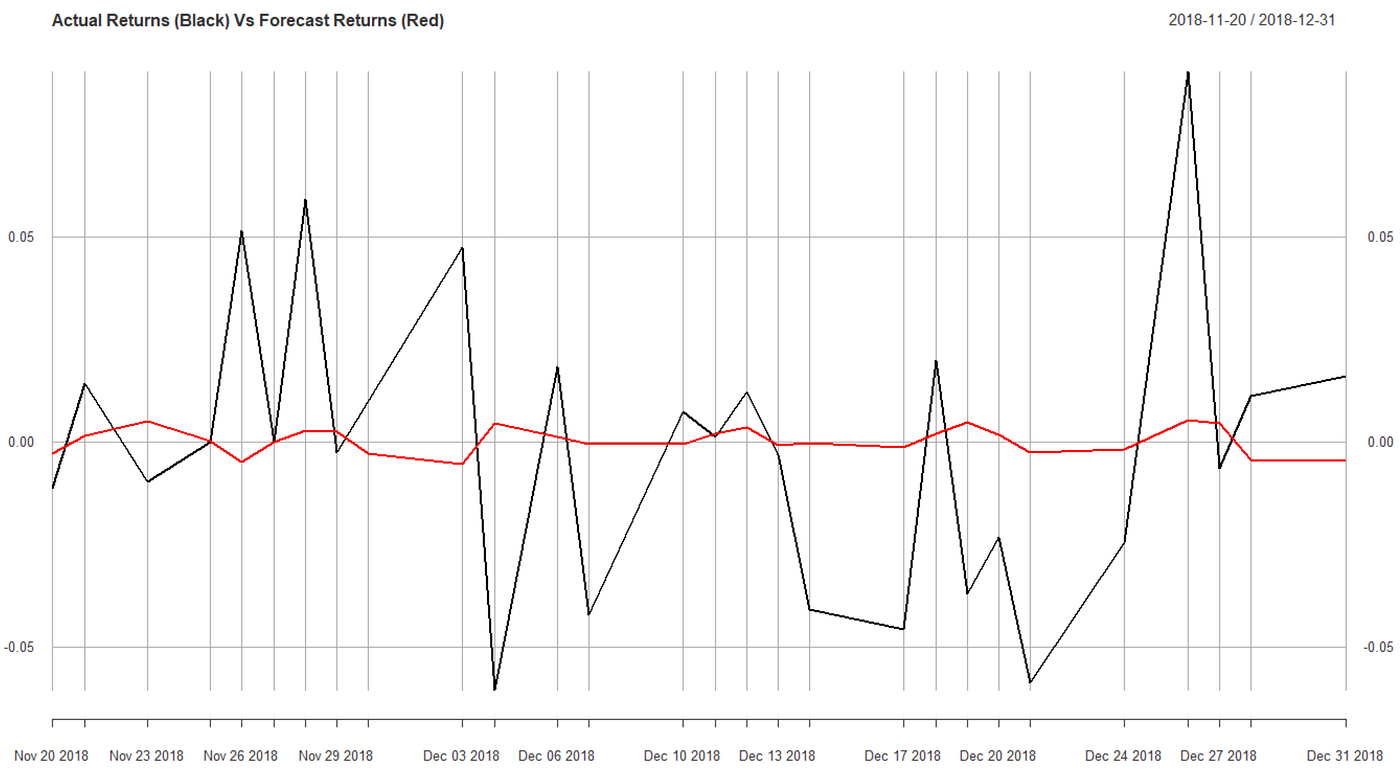This graph is specific to the AMZN stock returns over the testing period. The black line for actual returns serves as a benchmark for what happened in reality. The red line for forecast returns serves as the prediction for the increase or decrease in a return.

By viewing the red line, we can notice that the increases occur slightly before the trends occur in the black line. However, the model is not entirely accurate, since the fluctuations in the red line do not match the magnitude or frequency of those of the black line.

The following code merges the real and forecast return series from the testing sets, creates a binary comparison, and computes an accuracy percentage.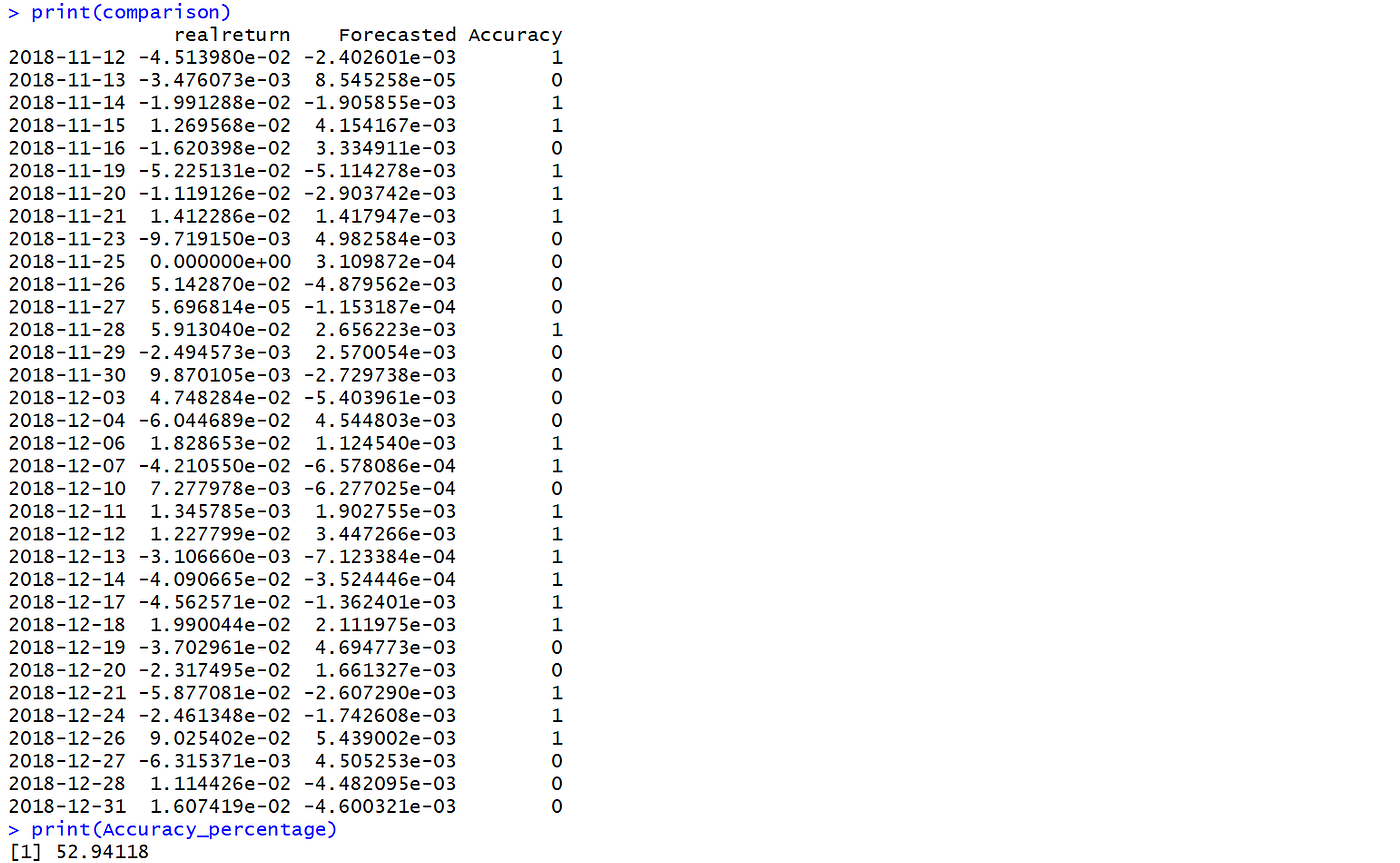The binary accuracy returns 1 if the signs computed from the program match and 0 if the signs computed from the program do not match. The accuracy percentage is the number of 1’s divided by the number of datapoints.

We now know that the model is 52.94% accurate when making a forecast of an increase or decrease in the logarithmic return of a stock.

# Conclusions And Interpretations

We talked a ton about statistics and plots and visualizing data, but more importantly, on a big-picture level, what does this all mean?

The AMZN stock value has grown over time, and if the trend continues, it should continue to grow. Based on the model, the values are likely to increase, as the forecast returns are more often above 0 than below.

The model built here is not strong enough to be used for investment strategies, but it can accurately tell us whether the stock value will increase or decrease in short-term intervals more than half of the time.We are slightly better than an uninformed investor at determining growth value based on this model, but in a world of accelerating and unpredictable change, the additional information can be a considerable factor for making better decisions.

Think of it this way. Instead of simply gambling on a stock for an increase in value, we now have a casino house advantage of about 2.94% based on the data.

Using an ARIMA model to forecast trends in a stock is not enough to make anyone a billionaire. After all, public sources such as walletinvestor.com provide accurate and detailed representations of stock predictions for free.

However, from this project, the main takeaway is the procedural structure with which the ARIMA model was built.

The statistical process that led to the ARIMA notation here is more rigorous than the R-shortcut command auto.arima().

The reason is that a model would be created regardless of whether the model would fit or not. In other words, we would have no idea whether we would need to smooth the data for a better model or whether the data was stationary. The extra checks allow for the model that does fit to be rigorously justified.

By implementing other statistical tests and modelling functions alongside the steps outlined in this project, a model that can predict more accurate returns can be designed.

Some suggestions for further coding are outlined below.

• The code is designed to be flexible with Yahoo Finance data, but adjustments could be made to extract non-public datasets, such as real-time data from Bloomberg Terminals.
• Though ARIMA can be performed on both seasonal and non-seasonal data, seasonal ARIMA, or SARIMA, would require a more complicated specification of the model structure.
• This process did not consider changes based on the model being additive or multiplicative, which would provide further insight on trends that would be smoothed.
• To better smooth prices, we can introduce weights for different combinations of seasonal, trend, and historical values to make predictions. We could link macroeconomic or industry factors at different points in time to show that growth or decline is justified.
• In addition to the Box-Ljung Test, we can also use the Breusch–Godfrey Test and the Durbin–Watson Test to improve rigorousness.
• The forecast model can be changed based on a confidence interval.
• As outlined in a paper by Selene Yue Xu, we can use weighted subjective opinions to combine technical analysis with fundamental analysis on a company.

Source References

# Acknowledgements

Many thanks to the University of British Columbia Department of Statistics for providing guidance for this project.

Additional credits go to the University of British Columbia Sauder School of Business Research Centres for providing Bloomberg terminals, ResearchGate access, and verified source material databases to enhance the scope of the research.

For RStudio source code, in-depth information or queries, feel free to comment below or email me (aaronjyen@gmail.com).

I am also looking for a role in Market Data or Business Analytics! If anyone has a position open, I’d love to add value to your business. Email me or message me for a chat or resume on my Linkedin (https://www.linkedin.com/in/aaronjyen/).

--

--## Aaron Yen

Independent financial analyst with a background in Statistics and Neuroscience. Currently researching market sectors for prospective clients and firms